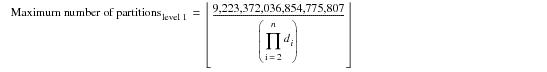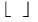# 15.00 - Determining the Maximum Number of Partitions for a Partitioned Database Object - Teradata Database

prodname
vrm_release
15.00
category
User Guide
featnum
B035-1094-015K

### Determining the Maximum Number of Partitions for a Partitioned Database Object

When you define a partitioned database object, the question of how many partitions to define per partitioning level must be addressed.

If the table or join index is defined with multiple partitioning levels, you should explicitly specify the number of partitions per partitioning level using the ADD option (see “CREATE TABLE” in SQL Data Definition Language). If you do not, Teradata Database reserves any excess partitions for partitioning level 1, and these partitions cannot be added to any other partitioning level once the table or join index is created.

The maximum number of partitions that are available for the level 1 partition from excess partitions depends on whether you have defined the object with 2‑byte partitioning or with 8‑byte partitioning.

The maximum number of partitions for level 1 for 2‑byte partitioning is the following.The maximum number of partitions for level 1 for 8‑byte partitioning is the following.where:

 Equation element … Specifiesthe floor, or lower truncated value, of the expression. di the number of partitions defined at level i.
• For a column‑partitioning level, if
• You do not specify an ADD clause for the column-partitioning level
• There is also row partitioning
• AND

• At least one of the row-partitioning levels does not specify an ADD clause
• Then the maximum number of partitions for the column-partitioning level is the number of column partitions defined plus 10.

• For a column‑partitioning level, if
• You do not specify an ADD clause for the column-partitioning level
• There is also row partitioning
• All of the row-partitioning levels specify an ADD clause
• AND

• Using the number of column partitions defined plus 10 as the maximum number of column partitions, the partitioning would be 2-byte partitioning
• Then the maximum number of partitions for the column-partitioning level is the largest value that does not cause the partitioning to be 8-byte partitioning.

Otherwise, the maximum number of partitions for the column-partitioning level is the largest value that does not cause the maximum combined partition number to exceed 9,223,372,035,854,775,807.

For single‑level partitioning, the maximum combined partition number is the same as the maximum partition number for the partitioning level.

• For a column-partitioning level, the maximum partition number is 1 more than the maximum number of partitions for that level. This is so that at least 1 unused column partition number is always available for altering a column partition.
• For each row-partitioning level without an ADD clause in level order, the maximum number of partitions for the row-partitioning level is the largest value that does not cause the partitioning to be 8-byte partitioning if using the number of row partitions defined as the maximum for this and any lower row-partitioning level without an ADD clause the partitioning would be 2-byte partitioning, is the largest value that does not cause the partitioning to be 8-byte partitioning.
• Otherwise, the maximum number of partitions for this level is the largest value that does not cause the maximum combined partition number to exceed 9,223,372,035,854,775,807.

• The maximum number of partitions for a row-partitioning level is at least 2.
• Otherwise, Teradata Database aborts the request and returns an error to the requestor. This error occurs when only one partition is defined for a row-partitioning level with an ADD 0 or with no ADD option and the maximum is not increased to at least 2.

• Having a maximum number of partitions for a partitioning level that is larger than the number of defined partitions for that level might enable the number of defined partitions for that level to be increased using an ALTER TABLE request.
• The maximum number of partitions for a level cannot be increased.

• For a row-partitioning level, the maximum partition number is the same as the maximum number of partitions for that level.
• The maximum combined partition number is the product of the maximum partition number determined for each partitioning level. The value cannot exceed 9,223,372,036,854, 775,807; otherwise, Teradata Database aborts the request and returns an error to the requestor.
• For single-level partitioning, the maximum combined partition number is the same as the maximum partition number for the partitioning level.

• The number of combined defined partitions is the product of the number of defined partitions for each partitioning level.
• The maximum number of combined partitions is the product of the maximum number of partitions determined for each partitioning level.
• For single-level partitioning, the maximum number of combined partitions is the same as the maximum number of partitions for the partitioning level.

If there is column partitioning, the maximum number of combined partitions is smaller than the maximum combined partition number or it is the same.

• If the maximum combined partition number is greater than 65,535, the partitioning is 8‑byte partitioning; otherwise, it is 2-byte partitioning.
• Single-level column partitioning with no ADD option is 2-byte partitioning.

• The rules listed in the following table apply to 2‑byte partitioning and to 8‑byte partitioning for both row‑partitioned and column‑partitioned tables and join indexes.
•
 For this partitioning … The maximum number of partitions for the first level is increased to … And the maximum number of partitioning levels is … 2‑byte the largest value that does not cause the maximum combined partition number to exceed 65,535 if it does not already do so. If there is at least one level with an explicit ADD clause, there is at least one level that consists solely of a RANGE_N function with BIGINT data type, or there is column‑partitioning, this is repeated for each of the other levels, if any, from the second level to the last level. 15 8‑byte the largest value that does not cause the maximum combined partition number to exceed 9,223,372,036,854,775, 807 if it does not already do so. This is repeated for each of the other levels from the second level to the last level. 62

You can alter any level to have between 1 and the maximum number of partitions allowed for that partitioning level.